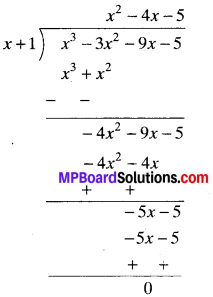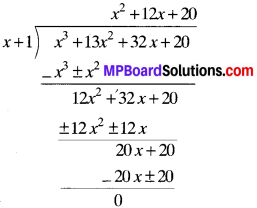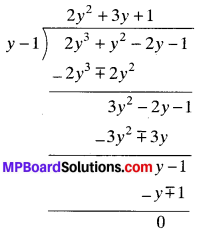## MP Board Class 9th Maths Solutions Chapter 2 Polynomials Ex 2.4

Question 1.
Determine which of the following polynomials has (x + 1) as factor:

1. x3 + x2 + x + 1
2. x4 + x3 + x2 + x + 1
3. x4 + 3x2 + 3x2 + x + 1
4. x3 – x2 – (2 + √2 ) x + √2

Solution:
1. p(x) = x3 + x2 + x + 1
x + 1 = 0 ∴ x = – 1
p(-1) = (-1)3 + (-1)2 + (-1) + 1
= – 1 + 1 – 1 + 1 = 0
(x + 1) is a factor of p(x).

2. p(x) = x4 + x3 + x2 + x + 1
x + 1 = 0, x = – 1
P(- 1) = (- 1)4 + (-1)3 + 3(-1)2 + (-1) + 1
= 1 – 1 + 1 – 1 + 1 = 1
(x + 1) is not a factor of p(x).

3. p(x) = x4 + 3x3 + 3x2 + x + 1
x + 1 = 0,
x = – 1
p(-1) = (-1)4 + 3(-1)3 + 3(-1)3 + (-1) + 1
= 1 – 3 + 3 – 1 + 1 = 1
(x + 1) is not a factor of p(x).

4. p(x) = x3 – x2 – (2 + √2 ) x + √2
x + 1 = 0, x = – 1
p(-1) = (-1)3 – (-1)2 – (2 + √2) x (-1) + √2
= – 1 – 1 + 2 + √2 + √2 = 2√2
(x + 1) is not a factor of p(x).Question 2.
Use the factor theorem to determine whether g(xr) is a factor of p(x) in each of the following cases:

1. p(x) = 2x3 + x2 – 2x – 1, g(x) = x + 1
2. p(x) = x3 + 3x2 + 3x + 1, g(x) = x + 2
3. p(x) = x2 – 4x2 + x + 6, g(x) = x – 3

Solution:
1. p(x) = 2x3 + x2 – 2x – 1, g(x) = 0
x + 1 = 0 x = – 1
p(-1) = 2(-1)3 + (-1)2 – 2(-1) – 1
= – 2 + 1 + 2 – 1 = 0
(x + 1) is a factor of p(x).

2. p(x) = x3 + 3x2 + 3x + 1, g(x) = x + 2
g(x) = 0
x + 2 = 0, x = – 2
zero of g(x) is – 2.
Now, p(-2)
= (-2)3 + 3 (-2)2 + 3(-2) + 1 ,
= – 8 + 12 – 6 + 1 = – 1 ≠ 0
By factor theorem, g(x) is not a factor of p(x).

3. p(x) = x3 – 4x2 + x + 6
g(x) = x – 3 = 0, x = 3
p(3) = (3)3 – 4(3)2 + (3) + 6
= 27 – 36 + 3 + 6
= 36 – 36 = 0
(x – 3) is a factor of p(x).

Question 3.
Find the value of k, if x – 1 is a factor of p(x) in each of the following cases:

1. p(x) = x2 + x + k
2. p(x) = 2x2 + kx + √2
3. p(x) = kx2 – √2x + 1
4. p(x) = kx2 – 3x + k.

Solution:

1. p(x) = x2 + x + k
If x – 1 is a factor of p(x), then p( 1) = 0
(∴ By factor theorem)
(1)2 + (1) + k = 0
1 + 1 + k = 0
2 + k = 0
k = – 2

2. p(x) = 2x2 + kx + √2
g(x) = x – 1, x = 1
P(1) = 2(1)2 + k(1) + √2 = 0
2 + k + √2 = 0
k = – (2 + √2)

3. p(x) = kx2 – √2 x + 1
g(x) = x – 1,
x = 1
p(1) = k(1)2 – √2(1) + 1
k – √2 + 1 = 0
k = √2 – 1

4. p(x) = kx2 – 3x + k
g(x) = x – 1, x = 1
p(1) = k(1)2 – 3(1) + k = 0
k – 3 + k = 0
2k – 3 = 0

Question 4.
Factorise:

1. 12x2 – 7x + 1
2. 2x2 + 7x + 3
3. 6x2 + 5x – 6
4. 3x2 – x – 4

Solution:
1. 12x2 – 7x + 1
12x2 – 7x + 1 = 12x2 – 4x – 3x + 1
= 4x(3x – 1) – 1 (3x – 1)
= (3x – 1) (4x – 1)

2. 2x2 + 7x + 3
2x2 + 7x + 3 = 2x2 + 6x + x + 3
= 2x (x + 3) + 1 (x + 3)
= (x + 3) (2x + 1)

3. 6x2 + 5x – 6
6x2 + 5x – 6 = 6x2 + 9x – 4x – 6
= 3x(2x + 3) – 2(2x + 3)
= (2x + 3) (3x – 2)

4. 3x2 – x – 4
3x2 – x – 4 = 3x2 – 4x + 3x – 4
= x(3x – 4) + 1(3x – 4)
= (3x – 4) (x + 1)Question 5.
Factorize:

1. x3 – 2x2 – x + 2
2. x3 – 3x2 – 9x – 5
3. x2 + 13x2 + 32x + 20
4. 2y3 + y2 – 2y – 1.

Solution:
1. x3 – 2x2 – x + 2
Let p(x) = x3 – 2x2 – x + 2
We find that
p(1) = (1)3 – 2(1)2 – (1) + 2
= 1 – 2 – 1 + 2 = 0
By factor theorem, (x – 1) is a factor of p(x).
Now,
x3 – 2x2 – x + 2 = x2(x – 1) – x(x – 1) – 2(x – 1)
= (x – 1) (x2 – x – 2)
= (x – 1) (x2 – 2x + x – 2)
= (x – 1) {x(x – 2) + 1(x – 2)}
= (x – 1) (x – 2) (x + 1).

2. p(x) = x3 – 3x2 – 9x – 5
x = – 1
p(-1) = (-1)3 – 3(-1)2 – 9(- 1) – 5
= – 1 – 3 + 9 – 5 = 0
(x + 1) is a factor of p(x).Now, p(x) = (x + 1) (x2 – 4x – 5)
= (x + 1) (A2 – 5A + A – 5)
= (x + 1) [x(x – 5) + 1 (x – 5)]
= (x + 1) (x – 5) (x + 1)

3. p(x) = x3 + 13x2 + 32x + 20
x = – 1,
p(-1) = (-1)3 + 13(-1)2 + 32(-1) + 20
= – 1 + 13 – 32 + 20 = 0
(x + 1) is a factor of p(x)p(x) = (x + 1) (x2 + 12x + 20)
= (x + 1) (x2 + 10x + 2x + 20)
= (x + 1) [x (x + 10) + 2 (x + 10)]
= (x + 1) (x + 2) (x + 10)

4. p(y) = 2y3 + y2 – 2y – 1
y = 1
P(1) = 2(1)3 + (1)2 – 2(1) – 1
= 2 + 1 – 2 – 1
= 0
(y – 1) is a factor of p(y)p(x) = (y – 1) (2y2 + 3y + 1)
= (y – 1) (2y2 + 2y + 1y + 1)
= (y – 1)[2y(y + 1) + 1 (y + 1)]
= (y – 1) (y + 1) (2y + 1)

Algebraic Identities:

1. (x + y)2 = x2 + 2xy + y2
2. (x – y)2 = x2 – 2xy + y2
3. x2 – y2 = (x + y) (x – y)
4. (x + a) (x + b) = x2 + (a + b) x + ab